EngliSea > M > math > 00 History

In mathematics, the notion of number has been extended over the centuries to include 0, negative numbers, rational numbers such as 1/2 and −2/3, real numbers such as √2 and π, and complex numbers, which extend the real numbers with √−1.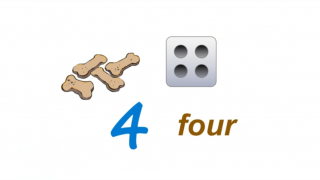number
A number is a count or measurement. It is really an idea in our minds. ○ count: the total determined by counting ○ measurement: the size, length or amount of something, as established by measuring
9577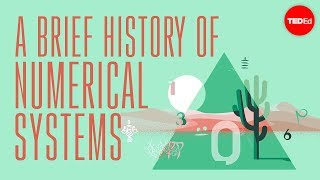0750± positional notation
By the 8th century, Indian mathematicians had perfected positional notation and over the next several centuries, Arab merchants, scholars and conquerors began to spread it into Europe. -- vigesimal, Maghreb, Hebrew, glyph, Aztecs, duodecimal, sexigesimal
4984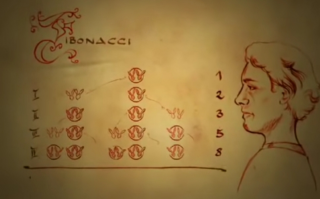1202 Leonardo Fibonacci
Fibonacci asked himself how many pairs of rabbits originated from a single pair in one year. Each pair of rabbits will produce exactly one more pair of both sexes per month which in turn would be fertile from the second month after birth.
4759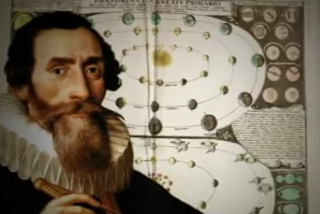1571 - 1630 Johannes Kepler
Keplar observed that the relationship between a number in Fibonacci sequence and the previous number more and more closely approaches the irrational number Φ the longer the sequence is continued and Φ describes nothing other than the golden section.
5056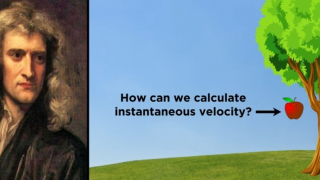1643 - 1727 Isaac Newton
Galileo had already discovered some years prior that the distance traveled by a falling object is represented by a function of time. Newton wondered how one could calculate the velocity of the object at any particular instance during the fall.
5035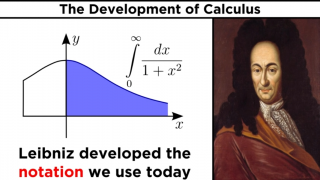1646 - 1716 Gottfried Wilhelm Leibniz
Leibniz did similar work just a few years later, independently of Newton, and it is actually his notation that we still use today.
5027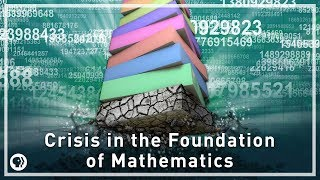1900± Crisis in Mathematics
Gottlob Frege, Bertrand Russel, Alfred North Whitehead, Zermelo-Fraenkel, Dedekind, Ernst Snapper
4835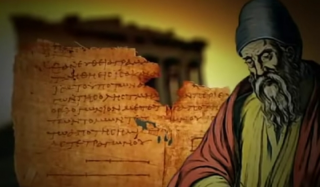BC 0300? Euclid
It was the Greek mathematician Euclid who produced the first precise description of the golden section. A length is devided into two parts in such a way that the smaller part is to the larger part in the same proportion as the larger one is to the whole.
5055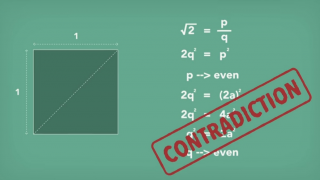BC 0500? Hippasus
According to Pythagoras theorem the diagonal length of a square with each side measuring one unit would be square root of two. The assumption that square root of two could be expressed as a ratio of two integers deduces a contradiction.
5026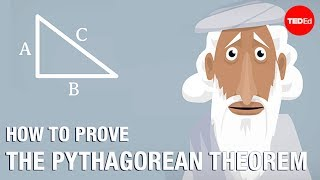BC 0570 - 0495 Pythagoras
○ How many ways are there to prove the Pythagorean theorem? - Betty Fei ○ James Garfeild, Pythagoras, hypotenuse, tessellation, Euclid
5039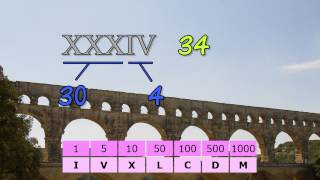BC 0800? Roman Numerals
The Romans showed numbers into the thousands using these 7 symbols: I for 1, V for 5, X for 10, L for 50, C for 100, D for 500 and M for 1000.
5164◌◌◌ dimension
The number of dimensions is how many values are needed to locate points on a shape.
9579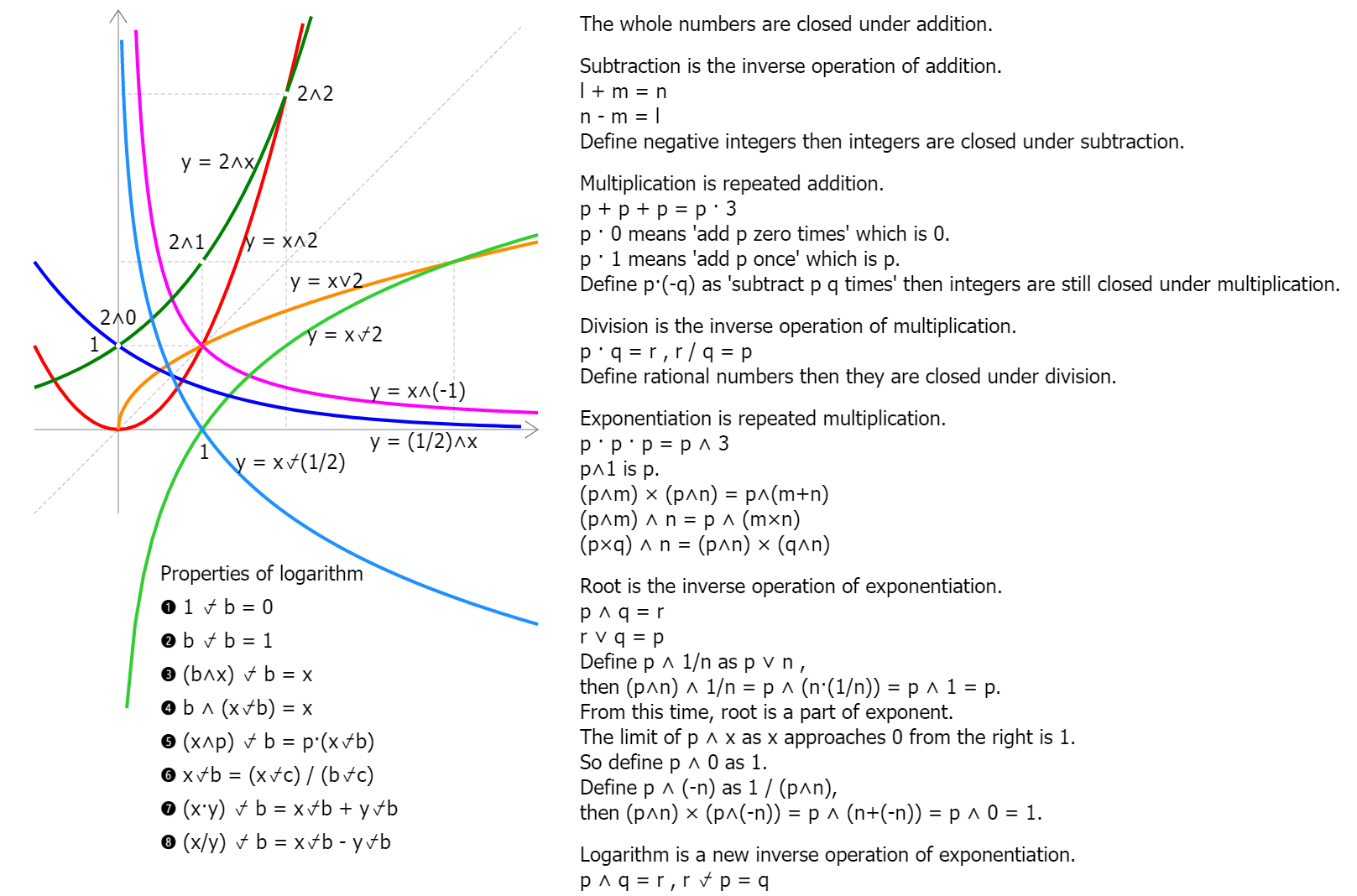Expansion of Numbers and Operations
9538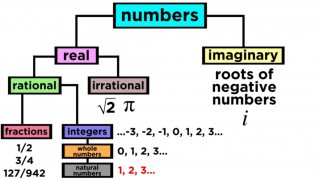Types of Numbers
a divine perfection, all hell broke loose, survey, taxonomy
9547-